# KR101267119B1 - Instrument and Method - Google Patents

## Info

Publication number
KR101267119B1
KR101267119B1 KR1020087022194 KR101267119B1 KR 101267119 B1 KR101267119 B1 KR 101267119B1 KR 1020087022194 KR1020087022194 KR 1020087022194 KR 101267119 B1 KR101267119 B1 KR 101267119B1
Authority
KR
South Korea
Prior art keywords
light
light intensity
intensity information
retarder
analysis target
Prior art date
Application number
KR1020087022194
Other languages
Korean (ko)
Inventor
유키토시 오타니
나오키 아사토
토시타카 와카야마
Original Assignee
고꾸리쯔 다이가꾸호우징 도쿄노우코우다이가쿠
Priority date (The priority date is an assumption and is not a legal conclusion. Google has not performed a legal analysis and makes no representation as to the accuracy of the date listed.)
Filing date
Publication date
Priority to JPJP-P-2006-00054153 priority Critical
Application filed by 고꾸리쯔 다이가꾸호우징 도쿄노우코우다이가쿠 filed Critical 고꾸리쯔 다이가꾸호우징 도쿄노우코우다이가쿠
Application granted granted Critical
Publication of KR101267119B1 publication Critical patent/KR101267119B1/en

## Images

•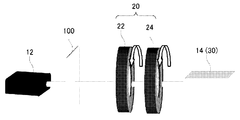•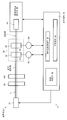•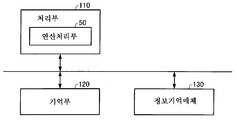•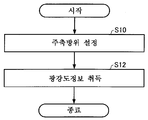••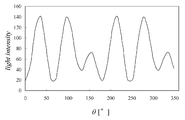•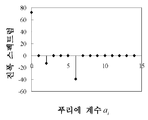•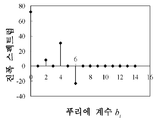•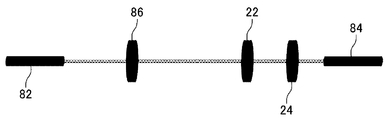•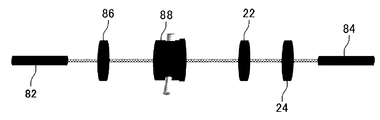•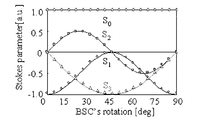•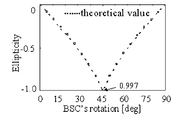•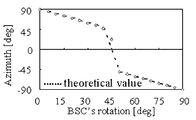•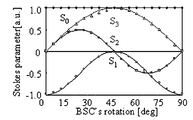•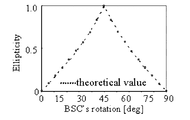••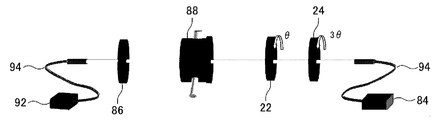•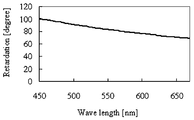•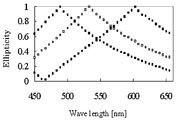•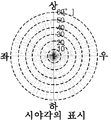••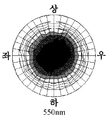•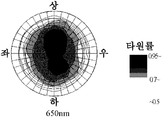## Abstract

The measuring device is a device for measuring the polarization state of the analysis target light to be analyzed, and modulates the analysis target light by the modulator 20 including the retarder 22 and the analyzer 24, and the modulator. And a light intensity information acquisition unit 30 for acquiring light intensity information of the modulated light obtained, and an arithmetic processing unit 50 for calculating the polarization characteristic element of the analysis target light based on the light intensity information. The light intensity information acquiring unit is a light of the first to Nth modulated light obtained by modulating the analysis target light in a modulator in which at least one of the main axis orientations of the retarder and the analyzer is set to the first to Nth main axis orientation conditions. Obtain strength information. The calculation processing unit performs polarization characteristic element calculation processing based on the theoretical formula of the light intensity of the first to Nth modulated light and the light intensity information of the first to Nth.
Liquid crystal, polarization, light intensity

## Description

MEASURING INSTRUMENT AND MEASURING METHOD}

The present invention relates to a measurement device for measuring the polarization state of the analysis target light, which is the object of analysis, and a measurement method for measuring the polarization state of the analysis target light.

In recent years, development of a new liquid crystal display material is actively performed. In connection with this, high precision is calculated | required also in the measuring method of product inspection. There is a circularly polarized film as a polymer film for liquid crystal display materials. This can compensate for narrow viewing angle deterioration due to birefringence and opticality of the liquid crystal and deterioration of the product due to coloration. In addition, since birefringence and linear light have wavelength dependence, evaluation for every wavelength is necessary. As the evaluation method, conventionally, the rotational analyzer method and the rotational displacement method have been used for ellipticity measurement. In addition, Japanese Patent Laid-Open No. 2005-292028 and R.M.A.Azaam, Ellipsometry and polarized light, (1976) are known as documents showing these techniques.

However, in the rotational analyzer method, since the ellipticity is given as an inverse sine function, the accuracy becomes poor when the birefringence phase difference of the measurement sample is about 90 degrees. In addition, in the rotational displacement method, it is necessary to replace the phase rulers in accordance with the wavelength to be measured, so that it is difficult to efficiently perform the evaluation for each wavelength.

An object of the present invention is to provide a measuring device and a measuring method which can analyze the polarization state of the analysis target light, which is the object of analysis, with high accuracy without exchanging the retarder for each wavelength.

(1) The measuring device according to the present invention,

As a measuring device for measuring the polarization state of the analysis target light to be analyzed,

A modulator configured to modulate the light to be analyzed, including a retarder and an analyzer configured to be rotatable;

A light intensity information acquisition unit for acquiring light intensity information of modulated light obtained by modulating the analysis target light in the modulator;

An arithmetic processing unit that performs arithmetic processing for calculating a polarization characteristic element of the analysis target light based on the light intensity information of the modulated light,

The modulator,

The light to be analyzed passes through the retarder, and is then configured to pass through the analyzer,

The light intensity information acquisition unit,

A first to Nth (N is an integer of 2 or more) satisfying a relationship in which the main axis bearing of the retarder and the main axis bearing of the analyzer are satisfied, and at least one of the main axis bearings of the retarder and the analyzer is different Obtains the light intensity information of the first to Nth modulated light obtained by modulating the light to be analyzed in the modulator set to the principal axis orientation condition of

The calculation processing unit,

The polarization characteristic based on a theoretical formula of the light intensity of the first to Nth modulated light reflecting the polarization characteristic element of the analysis target light and the principal axis orientation condition of the modulator and the light intensity information of the first to Nth modulated light. The process of calculating an element is performed.

In the measuring device according to the present invention, the theoretical formula of the light intensity of the modulated light acquired by the light intensity information acquisition unit reflects the polarization characteristic element of the analysis target light and the main axis orientation condition of the modulating unit. Therefore, by matching the theoretical formula of the light intensity of modulated light and the measured value, it becomes possible to calculate the polarization characteristic element of the analysis object light.

That is, according to this invention, it becomes possible to provide the measuring device which can analyze the polarization state of the analysis target light which is the object of analysis with high precision, without exchanging a phaser for every wavelength of the analysis target light. Moreover, according to this invention, since a measuring device can be comprised only by a simple drive system only by rotating a retarder and an analyzer, it becomes possible to provide the measuring device with high measurement efficiency and measurement accuracy.

The light intensity information of the modulated light can be obtained by analyzing the modulated light. The first to Nth main axis orientation conditions to be satisfied by the modulator can be selected in accordance with the analysis method of the light intensity information. Currently, various methods, such as a Fourier analysis method, are known as an analysis method of the light intensity information, but data suitable for analysis may vary depending on the analysis method. Therefore, in this invention, you may make it the setting which can acquire the data suitable for the analysis method which selects the 1st-Nth main axis orientation conditions.

However, if the main axis orientations of the retarder and the analyzer are θ 1 and θ 2 , θ 1 and θ 2 are 2θ 1 -2θ 2 ≠ 0, 4θ 1 -2θ 2 ≠ 0, and 2θ 1 -2θ 2 ≠ 4θ The condition of 1 -2θ 2 ? 2θ 2 may be satisfied, and accordingly, all elements of the Stokes parameter can be calculated by the Fourier analysis process.

The measuring device according to the present invention may be configured to include an optical system including a light source and a light receiving unit, and a modulating unit disposed on an optical path connecting the light source and the light receiving unit. At this time, the optical system may include a sample disposed between the light source and the modulator as an optical path. And the measurement apparatus may be comprised as a measurement apparatus which measures the optical characteristic element (birefringence phase difference, axle orientation, opticality, or Stokes parameter, a Muller matrix element, Jones matrix element, etc.) of a sample, and incident light incident on a sample By adjusting the polarization state of, it is possible to calculate these optical characteristic elements.

(2) The measuring device according to the present invention,

As a measuring device for measuring the polarization state of the analysis target light to be analyzed,

A light intensity information acquisition unit for acquiring light intensity information of the modulated light obtained by modulating the analysis target light in a modulator including a retarder and an analyzer configured to be rotatable;

An arithmetic processing unit that performs arithmetic processing for calculating a polarization characteristic element of the analysis target light based on the light intensity information of the modulated light,

The modulated light,

The analysis target light is light that has passed through the retarder, and then has passed through the analyzer;

The light intensity information acquisition unit,

1st to Nth (N is 2 or more) which satisfy | fills the relationship which the main-axis orientation of the retarder and the main-axis orientation of the said analyzer are, and at least one of the main-axis orientation of the said retarder and the said analyzer is different. Obtains the light intensity information of the first to Nth modulated light obtained by modulating the analysis target light in the modulator set to the principal axis orientation condition;

The calculation processing unit,

The polarization characteristic based on a theoretical formula of the light intensity of the first to Nth modulated light reflecting the polarization characteristic element of the analysis target light and the principal axis orientation condition of the modulator and the light intensity information of the first to Nth modulated light. The process of calculating an element is performed.

In the measuring device according to the present invention, the theoretical formula of the light intensity of the modulated light acquired by the light intensity information acquisition unit reflects the polarization characteristic element of the analysis target light and the main axis orientation condition of the modulating unit. Therefore, by matching the theoretical formula of the light intensity of modulated light and the measured value, it becomes possible to calculate the polarization characteristic element of the analysis object light.

That is, according to this invention, it becomes possible to provide the measuring device which can analyze the polarization state of the analysis target light which is the object of analysis with high precision, without exchanging a phaser for every wavelength of the analysis target light.

The light intensity information of the modulated light can be obtained by analyzing the modulated light. The first to Nth main axis orientation conditions to be satisfied by the modulator can be selected in accordance with the analysis method of the light intensity information. Currently, various methods, such as a Fourier analysis method, are known as an analysis method of the light intensity information, but data suitable for analysis may vary depending on the analysis method. Therefore, in this invention, you may make it the setting which can acquire the data suitable for the analysis method which selects the 1st-Nth main axis orientation conditions.

However, if the main axis orientations of the retarder and the analyzer are θ 1 and θ 2 , θ 1 and θ 2 are 2θ 1 -2θ 2 ≠ 0, 4θ 1 -2θ 2 ≠ 0, and 2θ 1 -2θ 2 ≠ 4θ The condition of 1 -2θ 2 ? 2θ 2 may be satisfied. According to this, it becomes possible to calculate all the elements of a Stokes parameter by a Fourier analysis process.

(3) In this measuring device,

If the main axis orientations of the retarder and the analyzer are set to θ 1 and θ 2 , respectively,

Kth principal axis orientation condition (K is each integer of 1 to N) of the modulator,

1 , θ 2 ) K = (180 × L × K / N, 180 × M × K / N)

(Where L and M are integers of 1 or more, and L ≠ M, L ≠ 2M, 2L ≠ M)

It may be.

As described above, the main axis orientations of the retarder and the analyzer are set at equal intervals, respectively, and the data of the retarder and the analyzer are changed to a band of 180 degrees or more (360 degrees or more), respectively. The analysis precision by a process (Fourier analysis process) can be improved.

In this measurement apparatus, L = 2, M = 6 and N = 30 may be used, for example. The main axis orientations of the retarder and the analyzer may be set to include the initial phase in the above conditions.

(4) The measuring device according to the present invention,

As a measuring device for measuring the polarization state of the analysis target light to be analyzed,

A modulator configured to modulate the light to be analyzed, including a retarder and an analyzer configured to be rotatable;

A light intensity information acquisition unit for acquiring light intensity information of the modulated light obtained by modulating the light to be analyzed in the modulator, wherein the retarder and the analyzer rotate at a given rotation rate;

An arithmetic processing unit that performs arithmetic processing for calculating the polarization characteristic element based on the light intensity information of the modulated light,

The modulator,

The light to be analyzed passes through the retarder, and is then configured to pass through the analyzer,

The light intensity information acquisition unit,

Acquires light intensity information of the modulated light whose intensity is continuously changed as analog information,

The calculation processing unit,

A process of calculating the polarization characteristic element is performed based on the theoretical formula of the light intensity of the modulated light reflecting the polarization characteristic element of the analysis target light and the principal axis orientation condition of the modulator and the light intensity information of the modulated light.

In the measuring device according to the present invention, the theoretical formula of the light intensity of the modulated light acquired by the light intensity information acquisition unit reflects the polarization characteristic element of the analysis target light and the main axis orientation condition of the modulating unit. Therefore, by matching the theoretical formula of the light intensity of modulated light and the measured value, it becomes possible to calculate the polarization characteristic element of the analysis object light.

That is, according to this invention, it becomes possible to provide the measuring device which can analyze the polarization state of the analysis target light which is the object of analysis with high precision, without exchanging a phaser for every wavelength of the analysis target light. Moreover, according to this invention, since the measuring device containing only a simple drive system only by rotating a retarder and an analyzer can be comprised, it becomes possible to provide the measuring device with high measurement efficiency and high measurement accuracy.

In addition, in the measuring device which concerns on this invention, the rotation ratio of a retarder and an analyzer can be selected according to the analysis method of light intensity information. That is, although the light intensity information of modulated light can be obtained by analyzing the modulated light, various methods, such as Fourier analysis method, are known as an analysis method of light intensity information, and data suitable for analysis varies according to the analysis method. There is a thing. Therefore, in this invention, you may set the rotation ratio of a retarder and an analyzer so that data suitable for the analysis method to select can be acquired.

The measuring device according to the present invention may include a light source and a light receiving unit, and include a optical system in which a modulator is disposed on an optical path connecting the light source and the light receiving unit. At this time, the optical system may include a sample disposed between the light source and the modulator as an optical path. And the measuring apparatus may be comprised as a measuring apparatus which measures the optical characteristic element (birefringence phase difference, axle orientation, or opticality, or Stokes parameter, a Muller matrix element, Jones matrix element, etc.) of a sample. By adjusting the polarization state of incident light incident on the sample, these optical characteristic elements can be calculated.

(5) The measuring device according to the present invention,

As a measuring device for measuring the polarization state of the analysis target light to be analyzed,

A light intensity information acquisition unit including a retarder and an analyzer, for acquiring light intensity information of the modulated light obtained by modulating the light to be analyzed in a modulator in which the retarder and the analyzer rotate at a given rotation ratio;

An arithmetic processing unit that performs arithmetic processing for calculating a polarization characteristic element of the analysis target light based on the light intensity information of the modulated light,

The modulated light,

The analysis target light is light that has passed through the retarder, and then has passed through the analyzer;

The light intensity information acquisition unit,

The light intensity information of the modulated light whose intensity is continuously changed is obtained as analog information,

The calculation processing unit,

A process of calculating the polarization characteristic element is performed based on the theoretical formula of the light intensity of the modulated light reflecting the polarization characteristic element of the analysis target light and the principal axis orientation condition of the modulator and the light intensity information of the modulated light.

In the measuring device according to the present invention, the theoretical formula of the light intensity of the modulated light acquired by the light intensity information acquisition unit reflects the polarization characteristic element of the analysis target light and the main axis orientation condition of the modulating unit. Therefore, by matching the theoretical formula of the light intensity of modulated light and the measured value, it becomes possible to calculate the polarization characteristic element of the analysis object light.

That is, according to this invention, it becomes possible to provide the measuring device which can analyze the polarization state of the analysis target light which is the object of analysis with high precision, without exchanging a phaser for every wavelength of the analysis target light.

In addition, in the measuring device which concerns on this invention, the rotation ratio of a retarder and an analyzer can be selected according to the analysis method of light intensity information. That is, although the light intensity information of modulated light can be obtained by analyzing the modulated light, various methods, such as Fourier analysis method, are known as an analysis method of light intensity information, and data suitable for analysis varies according to the analysis method. There is a thing. Therefore, in this invention, you may set the rotation ratio of a retarder and an analyzer so that data suitable for the analysis method to select can be acquired.

(6) In this measuring device,

The light intensity information acquisition unit,

The retarder and the analyzer may acquire the light intensity information of the modulated light obtained by modulating the light to be analyzed in the modulator that rotates so that the rotation ratio is 1: 3.

(7) In this measuring device,

The calculation processing unit,

You may perform the process which computes the said polarization characteristic element based on the some peak spectrum obtained by analyzing and processing the light intensity information acquired by the said light intensity information acquisition part, and the said theoretical formula.

At this time, as a method of analyzing the light intensity information, for example, DFT or FFT can be used.

(8) In this measuring device,

The calculation processing unit,

Prior to the processing for calculating the polarization characteristic element, the modulating unit modulates the sample light indicating a predetermined polarization state in place of the analysis target light, based on the light intensity information of the modulated light and the theoretical formula of the modulated light. Performing a birefringent phase difference calculation process for calculating the birefringent phase difference of the retarder,

You may perform the process which computes the said polarization characteristic element based on the birefringence phase difference of the said retarder computed by the said birefringence phase difference calculation process.

By using this measuring device, the birefringent phase difference of the retarder can be calculated. Therefore, if the birefringent phase difference of the retarder is calculated in advance, and the process of calculating the polarization characteristic element using this value, the calculation processing speed can be increased.

(9) In this measuring device,

The calculation processing unit may calculate a stock parameter of the analysis target light.

(10) In this measuring device,

The calculation processing unit may calculate at least one of an ellipticity of the analysis target light and a main axis orientation.

(11) In this measuring device,

First and second actuators for rotationally driving the retarder and the analyzer;

First and second detectors for detecting main axis orientations of the retarder and the analyzer;

And a control signal generator for generating a control signal for controlling the operation of the first and second actuators,

The control signal generator may generate the control signal based on detection signals from the first and second detectors.

(12) The measurement method according to the present invention,

As a measurement method of measuring the polarization state of the analysis target light to be analyzed,

A light intensity information acquisition procedure for acquiring light intensity information of modulated light obtained by modulating the analysis target light in a modulator including a retarder and an analyzer configured to be rotatable;

An arithmetic processing procedure for performing arithmetic processing for calculating a polarization characteristic element of the analysis target light based on the light intensity information of the modulated light;

The modulated light,

The analysis target light is light that has passed through the retarder, and then has passed through the analyzer;

In the light intensity information acquisition procedure,

Main axis bearings of the first to Nth (N is an integer of 2 or more) in which the main axis bearing of the retarder and the main axis bearing of the analyzer satisfy a given relationship, and at least one of the main axis bearings of the retarder and the analyzer is different. Acquires light intensity information of the first to Nth modulated light obtained by modulating the light to be analyzed in the modulator set as a condition;

In the arithmetic processing procedure,

The polarization characteristic based on a theoretical formula of the light intensity of the first to Nth modulated light reflecting the polarization characteristic element of the analysis target light and the principal axis orientation condition of the modulator and the light intensity information of the first to Nth modulated light. The process of calculating an element is performed.

In the measuring method concerning this invention, the theoretical formula of the light intensity of the modulated light acquired by the light intensity information acquisition part reflects the polarization characteristic element of an analysis object light, and the principal axis orientation condition of a modulating part. Therefore, by matching the theoretical formula of the light intensity of modulated light and the measured value, it becomes possible to calculate the polarization characteristic element of the analysis object light.

That is, according to this invention, it becomes possible to provide the measuring method which can analyze the polarization state of the analysis target light used as the analysis object with high precision, without exchanging a phase element for every wavelength of the analysis target light.

The light intensity information of the modulated light can be obtained by analyzing the modulated light. The first to Nth main axis orientation conditions to be satisfied by the modulator can be selected in accordance with the analysis method of the light intensity information. Currently, various methods, such as a Fourier analysis method, are known as an analysis method of the light intensity information, but data suitable for analysis may vary depending on the analysis method. Therefore, in this invention, you may make it the setting which can acquire the data suitable for the analysis method which selects the 1st-Nth main axis orientation conditions.

However, if the main axis orientations of the retarder and the analyzer are θ 1 and θ 2 , θ 1 and θ 2 are 2θ 1 -2θ 2 ≠ 0, 4θ 1 -2θ 2 ≠ 0, and 2θ 1 -2θ 2 ≠ 4θ 1 -2θ may be satisfies the condition of 2 ≠ 2θ 2. According to this, it becomes possible to calculate all the elements of a Stokes parameter by a Fourier analysis process.

(13) In this measuring method,

If the main axis orientations of the retarder and the analyzer are set to θ 1 and θ 2 , respectively,

Kth principal axis orientation condition (K is each integer of 1 to N) of the modulator,

1 , θ 2 ) K = (180 × L × K / N, 180 × M × K / N)

(Where L and M are integers of 1 or more, and L ≠ M, L ≠ 2M, 2L ≠ M)

It may be.

As described above, the main axis orientations of the retarder and the analyzer are set at equal intervals, and the retarder and the analyzer are changed to the bands of 180 degrees or more (360 degrees or more), respectively, thereby analyzing the data (fourier analysis). Processing) can improve the analysis accuracy.

In this measurement method, L = 2, M = 6 and N = 30 may be used, for example. In addition, the main axis orientations of the retarder and the analyzer may be set to include the initial phase in the above conditions.

(14) The measurement method according to the present invention,

As a measurement method of measuring the polarization state of the analysis target light to be analyzed,

A light intensity information acquisition procedure for acquiring light intensity information of modulated light obtained by modulating the light to be analyzed in a modulator in which a retarder and an analyzer rotate at a given rotation ratio,

An arithmetic processing procedure for performing arithmetic processing for calculating a polarization characteristic element of the analysis target light based on the light intensity information of the modulated light;

The modulated light,

The analysis target light is light that has passed through the retarder, and then has passed through the analyzer;

In the light intensity information acquisition procedure,

The light intensity information of the modulated light whose intensity is continuously changed is obtained as analog information,

In the arithmetic processing procedure,

A process of calculating the polarization characteristic element is performed based on the theoretical formula of the light intensity of the modulated light reflecting the polarization characteristic element of the analysis target light and the principal axis orientation condition of the modulator and the light intensity information of the modulated light.

In the measuring method concerning this invention, the theoretical formula of the light intensity of the modulated light acquired by the light intensity information acquisition part reflects the polarization characteristic element of an analysis object light, and the principal axis orientation condition of a modulating part. Therefore, by matching the theoretical formula of the light intensity of modulated light and the measured value, it becomes possible to calculate the polarization characteristic element of the analysis object light.

That is, according to this invention, it becomes possible to provide the measuring method which can analyze the polarization state of the analysis target light used as the analysis object with high precision, without exchanging a phase element for every wavelength of the analysis target light.

Moreover, in the measuring method which concerns on this invention, the rotation ratio of a retarder and an analyzer can be selected according to the analysis method of light intensity information. That is, the light intensity information of the modulated light can be obtained by analyzing the modulated light. Currently, various methods such as Fourier analysis are known as the analysis method of the light intensity information, and the data suitable for the analysis vary depending on the analysis method. There is a thing. Therefore, in this invention, you may set the rotation ratio of a retarder and an analyzer so that data suitable for the analysis method to select can be acquired.

(15) In this measuring method,

In the light intensity information acquisition procedure,

The light intensity information of the modulated light may be obtained by modulating the light to be analyzed in the modulator that rotates such that the retarder and the analyzer have a ratio of one to three.

(16) In this measuring method,

In the arithmetic processing procedure,

You may perform the process which computes the said polarization characteristic element based on the some peak spectrum obtained by analyzing and processing the light intensity information acquired by the said light intensity information acquisition procedure, and the said theoretical formula.

(17) In this measuring method,

Prior to the process of calculating the polarization characteristic element, the light intensity information of the modulated light obtained by modulating a sample light representing a predetermined polarization state in place of the analysis target light in the modulator, obtains the light intensity information and Further comprising a birefringent phase difference calculating process of calculating the birefringent phase difference of the retarder based on the theoretical formula of the modulated light,

In the arithmetic processing procedure,

You may perform the process which calculates the said polarization characteristic element based on the birefringence phase difference of the said retarder computed in the said birefringence phase difference calculation process order.

According to this measuring method, the birefringent phase difference of the retarder can be calculated. Therefore, if the birefringent phase difference of the retarder is calculated in advance, and the process of calculating the polarization characteristic element using this value, the calculation processing speed can be increased.

BRIEF DESCRIPTION OF THE DRAWINGS The figure for demonstrating the measuring apparatus which concerns on embodiment of this invention.

2 is a diagram for explaining a measurement device according to an embodiment of the present invention.

3 is a diagram illustrating an example of a functional block of an arithmetic processing system.

4 is a flowchart for explaining a light intensity information acquisition procedure.

5 is a flowchart for explaining a polarization characteristic element calculation procedure.

6 is a diagram illustrating an example of light intensity information.

7A is a diagram illustrating an example of light intensity information.

7B is a diagram illustrating an example of light intensity information.

8 is a diagram for explaining a verification experiment.

9 is a diagram for explaining a verification experiment.

10A is a diagram for explaining a verification experiment.

10B is a diagram for explaining a verification experiment.

10C is a diagram for explaining a verification experiment.

11A is a diagram for explaining a verification experiment.

11B is a diagram for explaining a verification experiment.

11C is a diagram for explaining a verification experiment.

12 is a diagram for explaining a verification experiment.

13 is a diagram for explaining a verification experiment.

14 is a diagram for explaining a verification experiment.

It is a figure which shows the result of the viewing angle characteristic evaluation of a circularly polarizing film.

It is a figure which shows the result of the viewing angle characteristic evaluation of a circularly polarizing film.

15C is a diagram showing results of evaluation of viewing angle characteristics of circularly polarized films.

It is a figure which shows the result of the viewing angle characteristic evaluation of a circularly polarizing film.

DESCRIPTION OF THE PREFERRED EMBODIMENTS Hereinafter, embodiments of the present invention will be described with reference to the drawings. In addition, embodiment described below is an example of this invention, and this invention is not limited to this. In addition, this invention shall include what combined the following contents freely.

EMBODIMENT OF THE INVENTION Hereinafter, the measuring device 1 which measures the polarization state of the light (analysis target light) radiate | emitted from the sample 100 as a measuring device which concerns on embodiment to which this invention is applied is demonstrated. In addition, the property of the sample 100 applicable to this invention is not specifically limited.

(1) device configuration

1 and 2 are diagrams for explaining the device configuration of the measurement device 1. 1 is a figure which shows typically the optical system 10 applicable to this invention (measuring apparatus 1), and FIG. 2 is a block diagram for demonstrating the structure of the measuring apparatus 1. As shown in FIG.

The measurement apparatus 1 includes an optical system 10, a light intensity information acquisition unit 30, and an arithmetic processing unit 50. The light intensity information acquisition unit 30 acquires light intensity information of the modulated light obtained by modulating the analysis target light (light modulated by the sample 100) in the modulator 20. That is, in the measuring device 1, the light intensity information acquisition unit 30 is emitted from the light source 12 and is modulated by the optical element and the sample 100 included in the optical system 10 (modulated light). Obtain the light intensity information of. In addition, in the measuring apparatus 1, the calculation processing part 50 is based on the theoretical formula of the light intensity of modulated light, and the optical characteristic of the light (analysis target light) modulated by the sample 100 based on the light intensity information of a modulated light. The process of calculating an element is performed. The sample 100 may be a material that transmits light or a material that reflects light.

Hereinafter, the apparatus structure of the measuring apparatus 1 is demonstrated.

1-1: Optical system 10

The optical system 10 includes a light source 12 and a light receiving portion 14. The optical system 10 further includes a retarder 22 and an analyzer 24 provided on an optical path L connecting the light source 12 and the light receiving portion 14. The retarder 22 and the analyzer 24 are optical elements which modulate the light (analysis target light) emitted from the sample 100. That is, the retarder 22 and the analyzer 24 are arrange | positioned downstream of the sample 100 in the optical path L. As shown in FIG. The retarder 22 and the analyzer 24 may be collectively referred to as a modulator 20. Hereinafter, each element of the optical system 10 will be described.

The optical system 10 includes a light source 12. The light source 12 is a device that generates and emits light. In the present embodiment, as the light source 12, an apparatus for emitting light containing a given wavelength (wavelength) band component may be used. For example, a white light source such as a halogen lamp may be used as the light source 12. The light source 12 may be a light source that emits light of a given wavelength (wavelength). At this time, the light source 12 can be said to be a light-emitting device which emits monochromatic light. As the light source 12, a laser, an SLD, or the like may be used. In addition, the light source 12 may have the structure which can change the wavelength (wavelength) of the light radiate | emitted.

The optical system 10 includes a retarder 22. The retarder 22 is an optical element whose magnitude of birefringence retardation differs depending on the wavelength of transmitted light. Therefore, the polarization state of the light transmitted through the retarder 22 changes depending on the wavelength. In addition, in the measuring device 1, the light incident on the retarder 22 (modulator 20) may be referred to as analysis target light. When the optical element is not disposed between the sample 100 and the retarder 22, the light emitted from the sample 100 may be referred to as an analysis target light. In the present invention, as the retarder 22, a primary retarder is used.

The optical system 10 includes an analyzer 24. The analyzer 24 is a polarizer on the emission side that linearly polarizes the light transmitted through the retarder 22 (light emitted from the retarder 22). In the optical system 10, light transmitted through the analyzer 24 (light emitted from the analyzer 24) enters the light receiving unit 14.

In the present invention, the retarder 22 and the analyzer 24 are collectively referred to as a modulator 20. And the retarder 22 and the analyzer 24 are comprised so that a main-axis orientation can be changed. The retarder 22 and the analyzer 24 may be comprised so that a main-axis orientation can be changed by rotating. In the measuring device 1, the light obtained by modulating the analysis target light in the modulator 20 is called modulated light.

The optical system 10 includes a light receiving unit 14. The light receiving unit 14 may be configured to receive light (modulated light) obtained by modulating the analysis target light in the modulator 20. As the light receiving portion 14, a CCD may be used, for example.

When the light to be analyzed (light modulated by the sample 100) is light including a given band component, the light receiving portion 14 may include a spectroscope and a plurality of light receiving elements. When the light to be analyzed is light including a given band component, the modulated light incident on the light receiving unit 14 also becomes light including the band component. At this time, by modulating the spectroscopic light for each wavelength and measuring the intensity of light of each wavelength in each light receiving element, the light intensity of the modulated light in a plurality of wavelength bands can be measured simultaneously.

In addition, a spectroscope is an optical device (optical element) which spectrographs light (for example, white light) containing a given band component for every wavelength. As a spectrometer, a prism or a diffraction grating can be used, for example. The light receiving element is an optical device (optical element) that measures the intensity of incident light by photoelectric conversion of incident light.

The optical system 10 may further include the polarizer 28 provided on the optical path L (refer FIG. 2). The polarizer 28 is arrange | positioned upstream of the sample 100 in the optical path L. As shown in FIG. That is, according to the optical system 10, the light emitted from the light source 12 is incident on the sample 100 through the polarizer 28, and the light modulated by the sample 100 is retarder 22 and It is comprised so that it may be made to enter into the light receiving part 14 through the analyzer 24 (modulator 20). That is, the light which modulated the light radiate | emitted from the light source 12 with the polarizer 28 and the sample 100 turns into analysis object light in the measuring device 1.

In addition, the measuring apparatus 1 which concerns on this embodiment is an apparatus which measures the polarization state of the light (analysis target light) which injects into the modulator 20 (retarder 22). Therefore, the structure of the upstream rather than the modulator 20 in the optical path L is not specifically limited. For example, in the measuring device 1, an optical system not including the polarizer 28 may be used. (See FIG. 1).

1-2: light intensity information acquisition unit 30

The light intensity information acquisition unit 30 acquires light intensity information of the modulated light. That is, the light intensity information acquisition unit 30 acquires light intensity information of light (modulated light) obtained by modulating the light (analysis target light) incident on the modulator 20 by the modulator 20. . In addition, the process which acquires the light intensity information of modulated light performed by the light intensity information acquisition part 30 may be called light intensity information acquisition process. The light intensity information acquisition unit 30 may be configured to acquire light intensity information of light incident on the light receiving unit 14. In addition, the light receiving unit 14 (spectrometer and light receiving element) may form part of the light intensity information acquisition unit 30.

In the measuring device 1, the light intensity information acquisition unit 30 satisfies a given relationship between the main axis orientation of the retarder 22 and the main axis orientation of the analyzer 24, and the retarder 22 and the sword. 1st thru | or 1st which is obtained by modulating the light to analyze in the modulation part 20 set at least one of the principal axis orientations of the photon 24 to the principal axis orientation conditions of another 1st-Nth (N is an integer of 2 or more) The light intensity information of N modulated light (plural modulated light) is obtained.

That is, the light intensity information acquisition unit 30 acquires light intensity information of the first to Nth (N is an integer of 2 or more), that is, N light intensity information. Here, the first to Nth light intensity information are light intensities of modulated light modulated by the modulator 20 set to the first to Nth main axis orientation conditions, respectively. In the first to Nth main axis orientation conditions, at least one main axis orientation setting of the optical element (the retarder 22 and the analyzer 24) is different from each other. In the first to Nth main axis orientation conditions, the main axis orientation of the retarder 22 and the main axis orientation of the analyzer 24 satisfy a predetermined relationship.

If the main axis orientation of the retarder 22 is θ 1 and the main axis orientation with the analyzer 24 is θ 2 , then the K-th main axis orientation condition is (θ 1 , θ 2 ) K = (180 × L × K / N, 180 × M × K / N) may be used. However, L and M are integers greater than or equal to 1 and satisfy L ≠ M. Further, L and M may be even. M may be an odd multiple of L (L is an odd multiple of M). For example, L = 2, M = 6 and N = 30 may be used. By setting θ 1 and θ 2 at equal intervals, and changing the retarder 22 and the analyzer 24 to the bands of 180 degrees or more (360 degrees or more), respectively, the analysis process of the data (Fourier analysis) Processing) can improve the analysis accuracy.

However, in the present invention, the modulator 20 does not necessarily satisfy the above-described main axis orientation condition. That is, in the present invention, since any of the known analysis methods can be applied, the light intensity information may be acquired under any of the main axis azimuth conditions in which data suitable for the selected analysis method can be obtained. . Alternatively, the main phase orientation of the retarder 22 and the main axis orientation of the analyzer 24 may be determined in consideration of the initial phase in the above-described main axis orientation conditions.

The plurality of light intensity information acquired by the light intensity information acquisition unit 30 may be stored in the storage device 40. The storage device 40 includes main axis orientation information (first to Nth main axis orientation conditions) of the modulator 20 (retarder 22 and analyzer 24), and first to Nth light intensities. Information may be stored in correspondence. And based on the light intensity information stored in the memory | storage device 40, the arithmetic processing part 50 performs the process which measured the polarization state of the analysis object light.

In the measurement device 1, the light intensity information acquisition unit 30 satisfies a given relationship between the main axis orientation of the retarder 22 and the main axis orientation of the analyzer 24, and further, the retarder 22. And light intensity information of a plurality of modulated lights obtained by modulating the analysis target light by the modulator 20 having at least one principal axis orientation of the analyzer 24 different from each other.

That is, the light intensity information acquisition unit 30 acquires light intensity information of the plurality of modulated lights. In addition, the said plurality of modulated light modulates the analysis target light by the modulator 20 which differs in the setting of at least one principal axis orientation of the optical element (retarder 22 and the analyzer 24), respectively. It is light obtained by. The plurality of modulated lights are light obtained by modulating the light to be analyzed by the modulator 20 in which the principal axis orientation of the retarder 22 and the principal axis orientation of the analyzer 24 satisfy a given relationship. It can be said.

1-3: arithmetic processing unit 50

The arithmetic processing unit 50 performs arithmetic processing for measuring the polarization state of the analysis target light. The calculation processing unit 50 performs processing (polarization characteristic element calculation processing) for calculating the polarization characteristic element of the analysis target light based on the theoretical formula of the light intensity of the modulated light and the light intensity information of the modulated light, and calculates the polarization state of the analysis target light. Measure it. Although described later in detail, the theoretical formula of the light intensity of modulated light includes the parameter which shows the polarization state of an analysis object light. Therefore, using the theoretical formula of the light intensity of modulated light and the light intensity information of modulated light, it becomes possible to calculate the parameter (polarization characteristic element) which shows the polarization state of an analysis object light. And when the polarization characteristic element of an analysis object light is computed, the polarization state of an analysis object light can be measured.

1-4: Drive / detection unit

The measurement device 1 may further include the first and second drive / detection sections 62 and 64. The drive part is an actuator which variablely set the main-axis orientation of the optical element which comprises an optical system among a drive / detection part. The detection unit is a sensor that detects the main axis orientation of the optical element. In the measuring device 1, the first drive / detector 62 rotates the retarder 22 to detect the main shaft orientation of the retarder 22. In addition, the second drive / detector 64 rotates the analyzer 24 to detect the main axis orientation of the analyzer 24.

And the measurement apparatus 1 may further include the control signal generation part 65 which controls the operation | movement of the 1st and 2nd drive / detection parts 62 and 64. As shown in FIG. For example, the control signal generation unit 65 generates a control signal based on the detection signals from the first and second driving and detection units 62 and 64, and the first and second driving and detection units ( 62 and 64 may be controlled to control the operation.

1-5: control device 70

The measuring device 1 may include the control device 70. The control device 70 may have a function of collectively controlling the operation of the measurement device 1. That is, the control apparatus 70 controls the 1st and 2nd drive / detection parts 62 and 64, sets the main-axis orientation of an optical element, controls the light emission operation of the light source 12, and light intensity The operations of the information acquisition unit 30 and the calculation processing unit 50 may be controlled.

The control device 70 may include a memory device 40 and an arithmetic processing unit 50. In addition, the storage device 40 has a function of temporarily storing various data. The memory device 40 may store the light intensity information of the modulated light in correspondence with the main axis orientation information of the retarder 22 and the analyzer 24, for example. And the arithmetic processing part 50 may perform the process of calculating the polarization characteristic element of the analysis object light based on the light intensity information stored in the memory | storage device 40. FIG. The control device 70 may further include a control signal generator 65.

In addition, the measurement apparatus 1 can perform the process using a computer especially in the control apparatus 70 (operation processing part 50). Here, a computer means a physical device (system) whose basic components are a processor (processing unit: CPU, etc.), a memory (memory unit), an input device, and an output device.

3 shows an example of a functional block of the arithmetic processing system constituting the control device 70.

The processing unit 110 performs various processes of the present embodiment based on the program (data) stored in the information storage medium 130. That is, the information storage medium 130 stores a program (a program for causing the computer to execute the processing of each part) for operating the computer as each part of the present embodiment.

The function of the processing unit 110 can be realized by hardware such as various processors (CPU, DSP, etc.), ASIC (gate array, etc.), and programs.

The storage unit 120 becomes a work area such as a processing unit, and its function can be realized by a RAM or the like.

The information storage medium 130 (medium that can be read by a computer) stores programs, data, and the like, and its functions are optical discs (CDs and DVDs), magneto-optical discs (MO), magnetic discs, hard discs, and magnetic fields. It can be implemented by a tape or a memory (ROM). In the measuring device 1, the main axis orientations of the modulator 20 (retarder 22 and analyzer 24) are set based on the program stored in the information storage medium 130, and the light source 12 is set. The light emission operation may be controlled.

(2) measurement principle of polarization characteristics

Next, the principle (the principle of calculating a polarization characteristic element) which measures the polarization state which the measuring apparatus which concerns on this embodiment employ | adopts is demonstrated.

2-1: Theoretical formula of light intensity of modulated light

The muller matrix R of the retarder 22 and the muller matrix A of the analyzer 24 are

Can be displayed as

Note that δ (λ), θ 1 , and θ 2 are the birefringent phase differences of the retarder 22, the rotation angle (main axis orientation) of the retarder 22, and the rotation angle (main axis orientation) of the analyzer 24. . In addition, since the birefringent phase difference of the retarder 22 has a wavelength dependency, it becomes a function of wavelength (lambda). That is, equation (1) is a birefringent phase difference of the retarder 22 when the wavelength? Light is incident on the retarder 22.

The polarization state S in (stock parameters) of the light (analysis target light) after exiting the sample 100,

When expressed as, S out which is the polarization state of modulated light obtained by modulating the analysis target light in the modulator 20,

Can be displayed as

In addition, s 0 (λ) in S in is light intensity, s 1 (λ) is linearly polarized light component, s 2 (λ) is 45 degree polarized light component, and s 3 (λ) is vector amount of circularly polarized light component. . S 0 (λ) in S out is a light intensity component of light (modulated light) incident on the light receiving portion 14. The light intensity I (λ, θ 1 , θ 2 ) of the modulated light is given by equation (4),

As shown in FIG. In addition, I 0 (λ) shown in equation (5) is a proportional constant of light intensity.

By the way, the light intensity (I) (λ, θ 1 , θ 2) and a bias component cos2θ 2, sin2θ 2, sin ( 2θ 1 -2θ 2), cos (4θ 1 -2θ 2), sin (4θ 1 -2θ of The amplitude component in 2 ) is obtained from equation (5),

Can be displayed as

Therefore, using the formulas (6a) to (6f), the birefringent phase difference δ (λ) of the retarder 22 is

Can be displayed as

In addition, as will be described later, since the left sides of the formulas (6a) to (6f) can be calculated from the light intensity information, the birefringence phase difference δ (λ) is calculated by substituting these values into the formula (7). can do.

In addition, using Formulas (6a) to (6f), s 0 (λ), s 1 (λ), s 2 (λ), and s 3 (λ) which are Stokes parameters of the analysis target light are respectively,

Can be displayed as

Since each left side of (6a) to (6f) and the birefringent phase difference δ (λ) of the retarder 22 can be calculated, by substituting these values into the formulas (8a) to (8d), Each value of the Stokes parameter of the light to be analyzed can also be calculated.

The ellipticity ε (λ) and the main axis orientation φ (λ) of the analysis target light are determined using Stokes parameters.

Can be displayed as

According to the principle which this invention employ | adopts from the above, it can calculate the polarization characteristic element of the analysis object light of wavelength (lambda), and can measure the polarization characteristic (polarization state) of the analysis object light of wavelength (lambda). Able to know. That is, even when the retarder which shows wavelength dependence in birefringence phase difference is used, it turns out that the polarization characteristic measurement in all wavelengths of the analysis object light can be performed.

2-2: Use of measured values

A 0 (λ), a 2θ 2 (λ), a 4θ 1-2θ 2 (λ), and b 2θ 2 (λ), b 2θ 1-2θ 2 (λ), represented by the left side of the formulas (6a) to (6f), b 4θ1-2θ2 (λ) indicates a bias component, a cos component, and a sin component of light intensity. That is, Fourier coefficients. Therefore, these coefficients are

. That is, a 0 (λ), a 2θ 2 (λ), a 4θ 1-2θ 2 (λ), and b 2θ 2 (λ), b 1-2θ 2 (λ), which appear on the left side of formulas (6a) to (6f), ), b 4θ1-2θ2 (λ) can calculate the value as a numerical value using light intensity information (actual value of light intensity).

And using these values, each value of Stokes parameter can be calculated from Formula (7) and Formula (8a)-Formula (8d).

And if each value of Stokes parameter is used, ellipticity and axle orientation can be computed as numerical value from Formula (9a) and Formula (9b).

2-3: Spindle bearing condition that the retarder 22 and the analyzer 24 must satisfy

As described above, the theoretical formula of the light intensity of the modulated light can be expressed by Equation (5), wherein the main axis direction θ 1 of the retarder 22 and the main axis direction θ 2 of the analyzer 24 are set. In some cases, a situation may occur in which all Stokes parameters s 0 (λ), s 1 (λ), s 2 (λ), and s 3 (λ) cannot be calculated.

For example, in the theoretical formula of the light intensity of light modulated by a modulator in which the main axis orientation of the retarder 22 and the main axis orientation of the analyzer 24 satisfy the relationship of 2θ 1 -2θ 2 = 0, Equation (5) ) of claim 4 are zero, it is not even possible to measure the s 3 (λ) by treatment analysis of the light intensity obtained. That is, in order to measure s 3 (λ), it is necessary for the main axis orientation of the retarder 22 and the main axis orientation of the analyzer 24 to satisfy the condition that 2θ 1 −2θ 2 ≠ 0.

Similarly, in order to calculate all Stokes parameters, the main axis direction of the retarder 22 and the main axis direction of the analyzer 24 are 2θ 1 -2θ 2 ≠ 0, 4θ 1 -2θ 2 ≠ 0, and 2θ. 1 -2θ 2 ≠ 4θ needs to be to meet the 1 -2θ 2 ≠ 2θ 2.

That is, when the main axis orientation of the retarder 22 and the main axis orientation of the analyzer 24 satisfy the above-described relationship, all the stokes parameters can be calculated. Therefore, efficient measurement is attained.

Specifically, in the light intensity information acquisition unit 30, the main axis orientation θ 1 of the retarder 22 and the main axis orientation θ 2 of the analyzer 24 satisfy the relationship of 3θ 1 = θ 2 . The analysis unit 20 may modulate the light to be analyzed, thereby acquiring light intensity information of the modulated light. According to this, since the retarder 22 and the analyzer 24 can satisfy the above conditions, it becomes possible to calculate all Stokes parameters.

For example, the retarder 22 and the analyzer 24 may be rotated so that the rotation ratio is 1: 3, so that the light intensity information may be acquired at regular intervals. Thereby, the light intensity information which can calculate all the stokes parameters can be acquired efficiently.

(3) Measurement procedure

Next, the measurement procedure of the polarization state by the measurement apparatus which concerns on this embodiment is demonstrated.

4 and 5 show an operation flowchart of the measurement apparatus according to the present embodiment.

3-1: light intensity information acquisition procedure

4 is a flowchart of a light intensity information acquisition procedure.

In the light intensity information acquisition procedure, first, the main axis orientations of the retarder 22 and the analyzer 24 (modulator 20) are set (step S10).

In this state, light is emitted from the light source 12, and light (modulated light) modulated by the optical element and the sample 100 is received by the light receiving portion 14. Then, the light intensity information acquisition unit 30 acquires the light intensity information of the light (modulated light) received by the light receiving unit 14 (step S12).

In the light intensity information acquisition procedure, light intensity information (light intensity information of first to Nth modulated lights) of the plurality of modulated lights is acquired. Here, the 1st to Nth modulated light is the measurement light obtained by modulating the analysis target light in the modulator 20 set to the 1st to Nth main axis orientation conditions. That is, in the light intensity information acquisition procedure, the above-described step S10 and step S12 are performed a plurality of times while changing the main axis orientation setting of the optical element.

In detail, in the measuring device 1, first, the main axis orientation of the optical element is set under the first condition, and the first light intensity information is obtained. The above-described memory device 40 is stored in association with the first condition (spindle orientation information) and the first light intensity information. Subsequently, the main axis orientation of the optical element is set (changed) as the second condition to acquire the second light intensity information, and the storage device 40 corresponds to the second condition and the second light intensity information. To store it. Hereinafter, this operation may be repeated, and N main axis orientation information and N light intensity information may be acquired, and each may be corresponded and stored in the memory | storage device 40. FIG.

In addition, the main axis orientation of the optical element of the optical system may be set (changed) by operating the actuators of the driving / detecting units 62 and 64 by the control signal generating unit 65. In addition, the main-axis orientation information of the optical element of an optical system may be detected by a detection part, and may be based on the information programmed beforehand.

3-2: Operation Processing Sequence

5 is a flowchart of a calculation processing procedure. In the arithmetic processing procedure, the polarization characteristic elements of the analysis target light are calculated based on the light intensity information of the modulated light acquired in the light intensity information acquisition order and the theoretical formula of the modulated light.

In the arithmetic processing procedure, first, a 0 (λ), a 2 θ 2 (λ), a 4 θ 1-2 θ 2 (λ), and b 2 θ 2 (λ), based on equations (10) to (12), b 2 θ1-2θ2 (λ), b 4 calculates the respective values of θ1-2θ2 (λ) (step S20).

Next, based on equations (7) and (8a) to (8d), the birefringence phase difference δ (λ) of the retarder 22 and the stokes parameters s 0 (λ) and s 1 ( λ), s 2 (λ), and s 3 (λ) are calculated (step S22).

And based on Formula (9a) and Formula (9b), the ellipticity (epsilon) (epsilon) and main-axis orientation (phi) (lambda) of an analysis target light are computed using each value of the Stokes parameter of an analysis target light (step S24). .

By the above procedure, an ellipticity and a principal axis orientation which are polarization characteristic elements of an analysis object light can be calculated, and the polarization state of an analysis object light can be measured.

(4) Regarding the birefringence phase difference δ (λ) of the retarder 22

As described above, according to the measuring device 1, even when a birefringent phase difference is used with an unknown retarder, the birefringent phase difference δ (λ) of the retarder 22 can be calculated from equation (7). The stock parameter of the light to be analyzed can be calculated.

By the way, when the Stokes parameter of the analysis target light becomes S in = {s 0 (λ), s 1 (λ), s 2 (λ), s 3 (λ)} = {1, 0, 0, 1} The birefringence phase difference δ (λ) cannot be calculated using Equation (7). In order to avoid this situation, the birefringence phase difference δ (λ) of the retarder 22 may be calculated in advance, calibration data may be obtained, and measurement may be performed using this value.

Specifically, in place of the analysis target light, a sample light whose Stokes parameter is not {1, 0, 0, 1} is incident on the modulator 20 to perform the light intensity information acquisition process, and the obtained light intensity information and On the basis of the theoretical formula of light intensity (refer to Formula (7)), the process of calculating birefringence phase difference (delta) ((lambda)) as calibration data may be performed. Then, the birefringent phase difference δ (λ) calculated according to the present procedure is stored in the storage device 40, and the above-described polarization characteristic element is calculated using the birefringence phase difference δ (λ) stored in the memory device 40. You may perform a process.

In addition, since the birefringent phase difference δ (λ) becomes a value unique to the retarder 22, this is calculated once and stored in the storage device 40, whereby the operation of calculating the birefringent phase difference δ (λ) is performed. There is no need to do this, and the operational efficiency can be improved.

(5) Modifications

Hereinafter, the measurement apparatus which concerns on the modification of embodiment to which this invention is applied is demonstrated. In addition, also in this embodiment, the content already demonstrated shall apply as much as possible.

In the measuring device according to the present embodiment, the light intensity information acquisition unit 30 modulates the analysis target light in the modulation unit 20 in which the retarder 22 and the analyzer 24 rotate at a given rotation ratio. Obtains light intensity information of modulated light. According to this, as shown in FIG. 6, the light intensity information acquisition part 30 can acquire the light intensity information of the modulated light which intensity changes continuously as analog information.

As shown in FIG. 6, it can be understood that the light intensity is a function having a period. Therefore, if this is an analysis process (for example, a Fourier analysis process), a peak spectrum can be extracted as shown in FIG. If these peak spectra correspond to the theoretical formula of light intensity (the left side of the above-described formulas (6a) to (6f)), the stock parameters of the analysis target light can be calculated based on the formulas (8a) to (8d). Can be.

(6) Verification result

Verification experiments were conducted to confirm the measurement principle and precision of the present invention. Hereinafter, the result is shown.

First, short wavelength measurement using a 633 nm helium neon laser was performed. In this experiment, as shown in FIG. 8, between the light source 82 and the power meter 84 (the light receiving unit 14 and the light intensity information obtaining unit 30) of the helium neon laser, The retarder 22 and the analyzer 24 were disposed. In addition, the main-axis orientation of the polarizer 86 was set to 0 degree. Moreover, the retarder 22 was rotated every 12 degrees, and the retarder 22 and the analyzer 24 were rotated by 1 to 3 rotation ratio. As the retarder 22, a quarter-wave plate of a 633 nm helium neon laser was used.

According to this experiment, the birefringence of the retarder 22 was 90 degrees, the main axis orientation was -0.15 degrees, and the ellipticity was 0.1%.

Next, as shown in FIG. 9, the sample was actually put in and the ellipticity was measured. In addition, in this experiment, the cabinet-soleil compensator 88 was used as a sample. The cabinet-soleil compensator 88 is an optical element (device) capable of arbitrarily adjusting the birefringence phase difference. 10A-10C show the results of Stokes parameters, ellipticity, and major axis orientations measured in this experiment. In addition, this experiment was performed by changing the main-axis orientation of the cabinet-soleil compensator 88 every 5 degrees from 0 degree to 90 degree. In addition, the solid line and broken line of FIGS. 10A-10C are a theoretical value, and a plot point is a measurement result.

10A to 10C show that the results of the Stokes parameters and the ellipticity were the same as the theoretical values, and even when the birefringence of the sample was measured at 90 degrees, it was found that 0.3% was obtained. In addition, in the measurement result of a main-axis orientation, it is shift | deviated from the theoretical value in the vicinity of the rotation angle 45 degree of the cabinet-soleil compensator 88. FIG. This shift | offset | difference is thought to be occurring because it is 99.7% of circularly polarized light.

Next, in order to verify the necessity of replacing the retarder, the same experiment was carried out by changing the retarder. In this experiment, a quarter wave plate having a wavelength of 457 nm was used as the retarder 22. The quarter-wave plate with a wavelength of 457 nm has a birefringence of about 65 degrees for the light of 633 nm. Therefore, it can be judged whether it is possible to measure even when the modulation amount of the retarder is other than 90 degrees. The results of this experiment are shown in Figs. 11A to 11C.

11A to 11C, the Stokes parameters, the ellipticity, and the major axis orientation all obtained the same results as the theoretical values. From this, according to the present invention, it can be confirmed that the polarization characteristic can be measured without depending on the amount of phase modulation of the retarder 22. In addition, from the results of this experiment, even when a 633 nm quarter-wave plate is used using a light source having a wavelength of 457 nm, a prediction can be made that polarization characteristics can be measured.

Next, experiments using light in multiple wavelength bands were performed. 12 shows the experimental apparatus used for this experiment.

As shown in FIG. 12, a halogen lamp is used as the light source 92, light from the light source 92 is led to the optical fiber 94, and parallel light is produced by a collimating lens. As the measurement sample, similar to the short wavelength experiment, a barbette-soleil compensator 88 was used, and a mica plate was used as the retarder 22. In addition, a halogen lamp is a white light source which spreads to the wavelength range of 400 nm-800 nm. Halogen lamps generally have low light intensity in the short wavelength region of 400 nm to 440 nm and 700 nm to 800 nm. For this reason, the measurement wavelength range was 450 nm to 660 nm.

In the measurement, first, the birefringent phase difference of the retarder 22 is obtained, and calibration data is acquired. The calibration data is similar to the measurement by the helium neon laser, and the retarder 22 (mica board) transmits the light transmitted through the polarizer 86 by the experimental apparatus from which the cabinet-soleil compensator 88 is removed. Analyze the waveform of the phase intensity light intensity. The birefringence dispersion of the mica plate obtained from the calibration is shown in FIG. 13.

Next, the measurement sample (Barnet-soleil compensator 88) was actually put in, and the ellipticity in the multiple wavelength region was measured. In this experiment, the cabinet-soleil compensator 88 is installed at 45 degrees of the main axis orientation, and a circularly polarized state is created at an arbitrary wavelength. The birefringent phase difference of the cabinet-soleil compensator 88 was changed by sending a micrometer to shift the wavelength of the circularly polarized state. In Fig. 14, the results of varying the birefringence phase difference of the cabinet-soleil compensator by varying the micrometer are shown. The retarder 22 was rotated every 12 degrees. Plot points in the figure are taken every 5 nm.

In either result, an ellipticity of 99% or more was obtained. In order to obtain a result of 100% ellipticity, it is considered that the improvement is achieved by more precisely installing the cabinet-soleil compensator and rotating the detection system.

From this experiment result, it turns out that even if the light of a multiple wavelength range is used, it is not necessary to replace the retarder 22, and it is possible to measure an ellipticity with high precision.

In addition, this invention is not limited to embodiment mentioned above, A various deformation | transformation is possible. This invention includes the structure substantially the same as the structure demonstrated by embodiment (for example, a structure with the same function, method, and result, or a structure with the same objective and effect). In addition, this invention includes the structure which substituted the non-essential part of the structure demonstrated by embodiment. Moreover, this invention includes the structure which achieves the same effect as the structure demonstrated by embodiment, or the structure which can achieve the same objective. Moreover, this invention includes the structure which added a well-known technique to the structure demonstrated by embodiment.

For example, the modulator 20 (the retarder 22 and the analyzer 24) may be configured to be capable of changing the main axis orientation manually. In this case, the main shaft orientation information may be obtained by the detection unit, and various calculation processes may be performed.

In addition, in the above embodiment, arithmetic processing using a muller matrix has been described. However, in this invention, you may perform arithmetic processing using a Jones matrix.

Moreover, according to this invention, the polarization state of the analysis object light can be measured and made clear. Therefore, it can apply to the light whose polarization state is unknown, and can measure the polarization state. That is, the polarization state can be measured without being particular about the properties of the measurement sample. In other words, the polarization state can be measured without being limited to the configuration on the upstream side of the retarder 22 in the optical system 10 (optical path L).

Moreover, the measuring apparatus (measurement method) which concerns on this invention is a measuring apparatus which measures the optical characteristic element (birefringence phase difference, axle orientation, opticality, or a Stokes parameter, a Muller matrix element, Jones matrix element, etc.) of the sample 100. It may be configured as a (measuring method). By selecting the properties of the light source and the optical element disposed between the light source and the sample 100, the optical characteristic element of the sample 100 can be calculated.

INDUSTRIAL APPLICABILITY The present invention can be used for evaluation of organic polymer materials including liquid crystals and research and development of new materials. Moreover, the orientation state of a polymer is applicable also to quality control. The knowledge obtained from them becomes very effective for new materials.

15A to 15D show the results of evaluating the viewing angle characteristics of the circularly polarized film using the present invention. Here, FIG. 15A has shown the display model of viewing angle distribution. 15B-15D are the figures which show the measurement result of the ellipticity of the light (analysis object light) of wavelength 450nm, 550nm, and 650nm which emitted the measurement object (sample 100), respectively. In addition, in each figure, the gray level has shown the magnitude | size of an ellipticity.

According to the present invention, the viewing angle distribution of the ellipticity of the light (analysis target light) emitted from the measurement object can be detected as shown in Figs. 15B to 15D.

In addition, it is understood from the results shown in FIGS. 15B to 15D that the measurement target used in this experiment has different ellipticity viewing angle distributions depending on the wavelength. For example, referring to FIG. 15B, it can be seen that the ellipticity of the top, bottom, left and right with the wavelength of 450 nm is almost uniform. On the other hand, in FIG. 15D, it can be seen that at the wavelength of 650 nm, the ellipticity in the vertical direction is high, and the ellipticity in the left and right directions is low.

That is, according to this invention, as shown to FIG. 15B-15D, the ellipticity distribution (polarization state of the analysis target light) of a measurement object for every wavelength band can be measured efficiently and correctly.

## Claims (17)

1. As a measuring device for measuring the polarization state of the analysis target light to be analyzed,
A modulator configured to rotate the birefringence retardation having a wavelength dependence, wherein the birefringence phase difference is configured to be rotatable at a rotation ratio of a given integer ratio, and modulates the light to be analyzed;
A light intensity information acquisition unit for acquiring light intensity information of modulated light obtained by modulating the analysis target light by rotating the retarder and the analyzer at the rotation ratio in the modulator;
An arithmetic processing unit that performs arithmetic processing for calculating a polarization characteristic element of the analysis target light based on the light intensity information of the modulated light,
The modulator,
The light to be analyzed passes through the retarder, and is then configured to pass through the analyzer,
The light intensity information acquisition unit,
At least one of the retarder and the analyzer's main axis orientations obtained by modulating the light to be analyzed in the modulating section set to other first to Nth (N is an integer of 2 or more) main axis orientations; Obtaining light intensity information of the Nth modulated light,
The calculation processing unit,
The retarder based on a theoretical formula of the light intensity of the first to Nth modulated light reflecting the polarization characteristic element of the analysis target light and the principal axis orientation condition of the modulator and the light intensity information of the first to Nth modulated light. A process for calculating all Stokes parameters associated with the polarization characteristic elements by reflecting the wavelength dependence of the birefringence phase difference of.
2. As a measuring device for measuring the polarization state of the analysis target light to be analyzed,
A modulator comprising a retarder and an analyzer having a birefringence phase difference having a wavelength dependence, and configured to be rotatable at a rotation ratio of a given integer ratio, wherein the retarder and the analyzer rotate at the rotation ratio to modulate the analysis target light. A light intensity information acquisition unit for acquiring light intensity information of the modulated light obtained by the
An arithmetic processing unit that performs arithmetic processing for calculating a polarization characteristic element of the analysis target light based on the light intensity information of the modulated light,
The modulated light,
The analysis target light is light that has passed through the retarder, and then has passed through the analyzer;
The light intensity information acquisition unit,
At least one of the retarder and the analyzer's main axis orientations obtained by modulating the light to be analyzed in the modulating section set to other first to Nth (N is an integer of 2 or more) main axis orientations; Obtaining light intensity information of the Nth modulated light,
The calculation processing unit,
The retarder based on a theoretical formula of the light intensity of the first to Nth modulated light reflecting the polarization characteristic element of the analysis target light and the principal axis orientation condition of the modulator and the light intensity information of the first to Nth modulated light. A process for calculating all Stokes parameters associated with the polarization characteristic elements by reflecting the wavelength dependence of the birefringence phase difference of.
3. As a measuring device for measuring the polarization state of the analysis target light to be analyzed,
A modulator for modulating the light to be analyzed, wherein the birefringence phase difference is configured to be rotatable at a rotation ratio of a given integer ratio and includes a retarder and an analyzer having a wavelength dependency;
A light intensity information acquisition unit for acquiring light intensity information of modulated light obtained by modulating the light to be analyzed by the modulator, wherein the retarder and the analyzer rotate at the rotation ratio;
An arithmetic processing unit which performs arithmetic processing for calculating polarization characteristic elements based on the light intensity information of said modulated light,
The modulator,
The light to be analyzed passes through the retarder, and is then configured to pass through the analyzer,
The light intensity information acquisition unit,
Acquires light intensity information of the modulated light whose intensity is continuously changed as analog information,
The calculation processing unit,
The polarization is reflected by reflecting the wavelength dependence of the birefringence phase difference of the retarder based on the theoretical formula of the light intensity of the modulated light reflecting the polarization characteristic element of the analysis target light and the principal axis orientation condition of the modulator and the light intensity information of the modulated light. And a process for calculating all stokes parameters associated with the characteristic elements.
4. As a measuring device for measuring the polarization state of the analysis target light to be analyzed,
A modulator comprising a retarder and an analyzer having a birefringence phase difference having a wavelength dependence, and configured to be rotatable at a rotation ratio of a given integer ratio, wherein the retarder and the analyzer rotate at the rotation ratio to modulate the analysis target light. A light intensity information acquisition unit for acquiring light intensity information of the modulated light obtained by the
An arithmetic processing unit that performs arithmetic processing for calculating a polarization characteristic element of the analysis target light based on the light intensity information of the modulated light,
The modulated light,
The analysis target light is light that has passed through the retarder, and then has passed through the analyzer;
The light intensity information acquisition unit,
The light intensity information of the modulated light whose intensity is continuously changed is obtained as analog information,
The calculation processing unit,
The polarization is reflected by reflecting the wavelength dependence of the birefringence phase difference of the retarder based on the theoretical formula of the light intensity of the modulated light reflecting the polarization characteristic element of the analysis target light and the principal axis orientation condition of the modulator and the light intensity information of the modulated light. And a process for calculating all stokes parameters associated with the characteristic elements.
5. 5. The method according to any one of claims 1 to 4,
The calculation processing unit,
All the stocks associated with the polarization characteristic elements reflecting the plurality of peak spectra obtained by analyzing the light intensity information acquired by the light intensity information acquiring unit and the wavelength dependence of the birefringence phase difference of the retarder based on the above theoretical formula. A process for calculating a parameter is performed.
6. 5. The method according to any one of claims 1 to 4,
The calculation processing unit,
Prior to the process of calculating the Stokes parameter, the retarder is based on the light intensity information of the modulated light and the theoretical formula of the modulated light, which are converted into the analysis target light and modulate a sample light indicating a predetermined polarization state in the modulator. Performing a birefringence phase difference calculation process for calculating the birefringence phase difference of
A process of calculating all stox parameters associated with the polarization characteristic element by reflecting the wavelength dependence of the birefringence phase difference of the retarder based on the birefringence phase difference of the retarder calculated by the birefringence phase difference calculation process. Measuring device.
7. 5. The method according to any one of claims 1 to 4,
And a rotation ratio of the given integer ratio as a ratio of one to three.
8. 5. The method according to any one of claims 1 to 4,
And the calculation processing unit calculates at least one of an ellipticity and the main axis orientation of the analysis target light.
9. 5. The method according to any one of claims 1 to 4,
First and second actuators for rotationally driving the retarder and the analyzer;
First and second detectors for detecting main axis orientations of the retarder and the analyzer;
Further comprising a control signal generator for generating a control signal for controlling the operation of the first and second actuators,
And the control signal generator generates the control signal based on detection signals from the first and second detection units.
10. As a measurement method of measuring the polarization state of the analysis target light to be analyzed,
A modulator comprising a retarder and an analyzer having a birefringence phase difference having a wavelength dependence, and configured to be rotatable at a rotation ratio of a given integer ratio, wherein the retarder and the analyzer rotate at the rotation ratio to modulate the analysis target light. A light intensity information acquisition procedure for acquiring light intensity information of modulated light obtained by
An arithmetic processing procedure for performing arithmetic processing for calculating a polarization characteristic element of the analysis target light based on the light intensity information of the modulated light;
The modulated light,
The analysis target light is light that has passed through the retarder, and then has passed through the analyzer;
In the light intensity information acquisition procedure,
At least one of the retarder and the analyzer's main axis orientations obtained by modulating the light to be analyzed in the modulating section set to other first to Nth (N is an integer of 2 or more) main axis orientations; Obtaining light intensity information of the Nth modulated light,
In the arithmetic processing procedure,
The retarder based on a theoretical formula of the light intensity of the first to Nth modulated light reflecting the polarization characteristic element of the analysis target light and the principal axis orientation condition of the modulator and the light intensity information of the first to Nth modulated light. And a process for calculating all the Stokes parameters associated with the polarization characteristic elements by reflecting the wavelength dependence of the birefringence phase difference of.
11. As a measurement method of measuring the polarization state of the analysis target light to be analyzed,
A modulator comprising a retarder and an analyzer having a birefringence phase difference having a wavelength dependence, and configured to be rotatable at a rotation ratio of a given integer ratio, wherein the retarder and the analyzer rotate at the rotation ratio to modulate the analysis target light. A light intensity information acquisition procedure for acquiring light intensity information of modulated light obtained by
An arithmetic processing procedure for performing arithmetic processing for calculating a polarization characteristic element of the analysis target light based on the light intensity information of the modulated light;
The modulated light,
The analysis target light is light that has passed through the retarder, and then has passed through the analyzer;
In the light intensity information acquisition procedure,
The light intensity information of the modulated light whose intensity is continuously changed is obtained as analog information,
In the arithmetic processing procedure,
The polarization is reflected by reflecting the wavelength dependence of the birefringence phase difference of the retarder based on the theoretical formula of the light intensity of the modulated light reflecting the polarization characteristic element of the analysis target light and the principal axis orientation condition of the modulator and the light intensity information of the modulated light. A process for calculating all stokes parameters associated with characteristic elements is performed.
12. The method according to claim 10 or 11, wherein
In the arithmetic processing procedure,
All the peak spectra obtained by analyzing the light intensity information acquired in the light intensity information acquisition order, and the wavelength dependence of the birefringence phase difference of the retarder based on the above theoretical formula, all of which are associated with the polarization characteristic element. A measurement method for calculating a stokes parameter.
13. The method according to any one of claims 10 to 11,
Prior to the process of calculating the Stokes parameter, the light intensity information of the modulated light obtained by modulating a sample light indicating a predetermined polarization state by converting it into the analysis target light and displaying a predetermined polarization state is obtained, and the light intensity information and the modulation are obtained. Further comprising a birefringent phase difference calculation process for calculating the birefringent phase difference of the retarder based on a theoretical formula of light,
In the arithmetic processing procedure,
A process for calculating all stokes parameters associated with the polarization characteristic elements by reflecting the wavelength dependence of the birefringence phase difference of the retarder on the basis of the birefringence phase difference of the retarder calculated in the birefringence phase difference calculation processing procedure. Measurement method to assume.
14. The method according to any one of claims 10 to 11,
The rotation ratio of said given integer ratio is set to rotation ratio of 1 to 3, The measuring method characterized by the above-mentioned.
15. delete
16. delete
17. delete
KR1020087022194 2006-02-28 2007-02-16 Instrument and Method KR101267119B1 (en)

## Priority Applications (1)

Application Number Priority Date Filing Date Title
JPJP-P-2006-00054153 2006-02-28

## Publications (1)

Publication Number Publication Date
KR101267119B1 true KR101267119B1 (en) 2013-05-23

# Family

## Citations (2)

* Cited by examiner, † Cited by third party
Publication number Priority date Publication date Assignee Title
JP2005241406A (en) 2004-02-26 2005-09-08 Nokodai Tlo Kk Instrument and method for measuring double refraction dispersion
JP2005257508A (en) 2004-03-12 2005-09-22 Nokodai Tlo Kk Double refraction characteristic measuring device and double refraction characteristic measuring method

## Patent Citations (2)

* Cited by examiner, † Cited by third party
Publication number Priority date Publication date Assignee Title
JP2005241406A (en) 2004-02-26 2005-09-08 Nokodai Tlo Kk Instrument and method for measuring double refraction dispersion
JP2005257508A (en) 2004-03-12 2005-09-22 Nokodai Tlo Kk Double refraction characteristic measuring device and double refraction characteristic measuring method

## Similar Documents

Publication Publication Date Title
US5337146A (en) Diffraction-grating photopolarimeters and spectrophotopolarimeters
Berry et al. Measurement of the Stokes parameters of light
EP1095259B1 (en) Spectroscopic ellipsometer
Goldstein Mueller matrix dual-rotating retarder polarimeter
US5872630A (en) Regression calibrated spectroscopic rotating compensator ellipsometer system with photo array detector
US6469788B2 (en) Coherent gradient sensing ellipsometer
US6816261B2 (en) Polarization analysis unit, calibration method and optimization therefor
JP4250894B2 (en) Optical encoder and encoder scale
US9581430B2 (en) Phase characterization of targets
Oka et al. Spectroscopic polarimetry with a channeled spectrum
Snik et al. Spectral modulation for full linear polarimetry
US6084675A (en) Adjustable beam alignment compensator/retarder with application in spectroscopic ellipsometer and polarimeter systems
JP5248722B2 (en) Surface characteristic analysis system with self-calibration function
US4589776A (en) Method and apparatus for measuring optical properties of materials
EP2583300B1 (en) Discrete polarization scatterometry
CA1229499A (en) Ellipsometric method and apparatus
US6081337A (en) Method and apparatus for measuring liquid crystal cell properties
EP0176827B1 (en) Method and apparatus for simultaneously determining gauge and orientation of polymer films
US6128080A (en) Extended range interferometric refractometer
JPH10339668A (en) Light wavemeter and light wavelength regulator
Todorov et al. Spectrophotopolarimeter: fast simultaneous real-time measurement of light parameters
KR19990076790A (en) 8 polarization modulating optical components, a Müller polarimeter and an integrator meter including such an optical component, a process for calibrating the integrator meter, and an integrator metering process
US5045701A (en) Infrared spectropolarimeter
JP4455024B2 (en) Birefringence measuring device
US6985227B2 (en) Birefringence measurement at deep-ultraviolet wavelengths